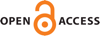Download this articleFor screen For printingRecent IssuesThe Journal About the Journal Editorial Board Editors’ Interests Subscriptions Submission Guidelines Submission Form Policies for Authors Ethics Statement ISSN: 1948-206X (e-only) ISSN: 2157-5045 (print) Author Index To Appear Other MSP JournalsA structure theorem for elliptic and parabolic operators with applications to homogenization of operators of Kolmogorov type

### Malte Litsgård and Kaj Nyström

Vol. 16 (2023), No. 7, 1547–1588##### Abstract

We consider the operators

 ${\nabla }_{X}\cdot \left(A\left(X\right){\nabla }_{X}\right),\phantom{\rule{1em}{0ex}}{\nabla }_{X}\cdot \left(A\left(X\right){\nabla }_{X}\right)-{\partial }_{t},\phantom{\rule{1em}{0ex}}{\nabla }_{X}\cdot \left(A\left(X\right){\nabla }_{X}\right)+X\cdot {\nabla }_{Y}-{\partial }_{t},$

where $X\in \mathrm{\Omega }$, $\left(X,t\right)\in \mathrm{\Omega }×ℝ$ and $\left(X,Y,t\right)\in \mathrm{\Omega }×{ℝ}^{m}×ℝ$, respectively, and where $\mathrm{\Omega }\subset {ℝ}^{m}$ is an (unbounded) Lipschitz domain with defining function $\psi :{ℝ}^{m-1}\to ℝ$ being Lipschitz with constant bounded by $M$. Assume that the elliptic measure associated to the first of these operators is mutually absolutely continuous with respect to the surface measure $d\sigma \left(X\right)$ and that the corresponding Radon–Nikodym derivative or Poisson kernel satisfies a scale-invariant reverse Hölder inequality in ${L}^{p}$, for some fixed $p$, $1, with constants depending only on the constants of $A$, $m$ and the Lipschitz constant of $\psi$, $M$. Under this assumption we prove that the same conclusions are also true for the parabolic measures associated to the second and third operators with $d\sigma \left(X\right)$ replaced by the surface measures $d\sigma \left(X\right)dt$ and $d\sigma \left(X\right)dYdt$, respectively. This structural theorem allows us to reprove several results previously established in the literature, as well as to deduce new results in, for example, the context of homogenization for operators of Kolmogorov type. Our proof of the structural theorem is based on recent results established by the authors concerning boundary Harnack inequalities for operators of Kolmogorov type in divergence form with bounded, measurable and uniformly elliptic coefficients.

##### Keywords
Kolmogorov equation, elliptic, parabolic, ultraparabolic, hypoelliptic, operators in divergence form, Dirichlet problem, Lipschitz domain, doubling measure, elliptic measure, parabolic measure, Kolmogorov measure, $A_{\infty}$, Lie group, homogenization
##### Mathematical Subject Classification
Primary: 35K65, 35K70, 35H20, 35R03
##### Milestones